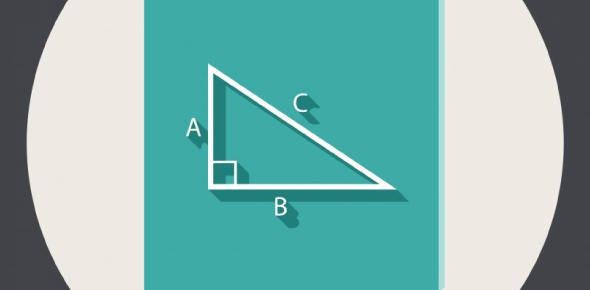Which of the following cannot be X? A triangle has sides of length 7, - ProProfs Discuss+ Ask Question

# Which of the following cannot be X? A triangle has sides of length 7, 11, and X.Change Image    Delete

A. 2
B. 4
C. 8
D. 12
E. 18

This question is part of sat math questions i

Asked by Harrison, Last updated: Jan 15, 2021

###Request 0FollowShareAnswer AnonymouslyAnswer LaterCopy LinkAnnJ

18 is also impossible, because the sum of two lenghts cannot be equal with the third, that means that the angle between sides 7 and 11 is 180, and that makes it... a line.The answer is clearly 2- if one side is 11 and the other is 7 the 3rd side must be long enough to reach look at the possible length of the 3rd side- if the 7 is on one side of the 11 side and the x is on the other if x were 2 then even with angels of 0 degrees the 7 side would not connect with the x side
​to make a triangle the sum of the 2 smaller sides must equal more than the largest side
7+2 <11John Smith2
The sum of lengths of two sides of a triangle cannot be less than the length of the third side. So, 2 + 7 = 9, but 9 < 11Search for Google imagesSelect a recommended image
Upload from your computerCancelSearch for Google imagesSelect a recommended image
Upload from your computerCancelSearch for Google imagesSelect a recommended image
Upload from your computerCancel